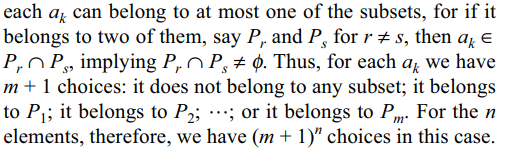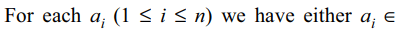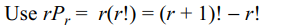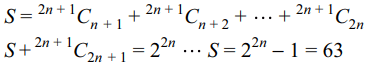## Permutations and Combinations Questions and Answers Part-7

1.Let $E=\left[\frac{1}{3}+\frac{1}{50}\right]+\left[\frac{1}{3}+\frac{2}{50}\right]+....$         upto 50 terms, then
a) E is divisible by exactly 2 primes
b) E is prime
c) $E\leq 35$
d) Both b and c

Explanation:2. If n < p < 2n and p is prime and $N=^{2n}C_{n}$    , then
a) $p\mid N$
b) $p^{4}\mid N$
c) $p^{2}\mid N$
d) $p^{3}\mid N$

Explanation: n! N = (n + 1) (n + 2) .... (2n)3. A is a set containing n elements. A subset $P_{1}$ of A is chosen. The set A is reconstructed by replacing the elements of $P_{1}$ . Next a subset $P_{2}$ of A is chosen and again the set is reconstructed by replacing the elements of $P_{2}$ . In this way m(> 1) subsets $P_{1},P_{2},....P_{m}$   of A are chosen. The number of ways of choosing $P_{1},P_{2},....P_{m}$   so that $P_{i}\cap P_{j}=\phi$    for $i\neq j$  , is
a) $\left(m+1\right)^{n}$
b) $2^{m}- ^{m}C_{n}$
c) $\sum_{k=0}^{n}.^{m}C_{k}m^{k}$
d) Both a and c

Explanation: Let the set A be {a1, a2, .... , an}. In the first case,4. With $P_{1},P_{2},....P_{m}$    as in Q3, the number of ways of choosing $P_{1},....P_{m}$   so that $P_{1}\cap P_{2}\cap....\cap P_{m}=\phi$     is
a) $\left(2^{m}-1\right)^{n}$
b) $\left(1+2+....+2^{m-1}\right)^{n}$
c) $\left(m+1\right)^{n}$
d) Both a and b

Explanation:5. The number of 10 digit numbers that can be formed by using the digits 2 and 3 is
a) $^{10}C_{2}+^{9}C_{2}$
b) $2^{10}$
c) $2^{10}-2$
d) 10!

Explanation: For each place we have two choices

6. If $P_{r}$ stands for $^{r}P_{r}$   , then sum of the series $1+P_{1}+2P_{2}+3P_{3}+....+nP_{n}$      is
a) $P_{n+1}$
b) $P_{n+1}-1$
c) $P_{n+1}+1$
d) none of these

Explanation:7. If $a_{n}=\sum_{r=0}^{n}\frac{1}{^{n}C_{r}}$    then value of$\sum_{r=0}^{n}\frac{n-2r}{^{n}C_{r}}$     is
a) $\frac{n}{2}a_{n}$
b) $\frac{1}{4}a_{n}$
c) $na_{n}$
d) 0

Explanation:8. m men and w women are to be seated in a row so that all men sit together. The number of ways in which they can be seated is
a) (w + 1)! m!
b) m! w!
c) m! (w – 1)!
d) $^{n}C_{w}$

Explanation: First treat m men as just one object. We can permute (w + 1) objects in (w + 1)! and the m men is m! ways.

9. A five digit number divisible by 6 is to be formed by using the digits 0, 1, 2, 3, 4 and 8 without repetition.The total number of ways in which this can be done is
a) 216
b) 150
c) 116
d) 98